### Home > PC > Chapter 9 > Lesson 9.3.4 > Problem9-145

9-145.
1. Let f(x) = x3. Homework Help ✎

1. Find the average rate of change from x = 3 to x = 5.

2. Show that the average rate of change from x = 4 to x = 4 + h is 48 + 12h + h2.

3. Find f ′(4), the instantaneous rate of change when x = 4.

4. Show that the average rate of change from x to x + h is 3x2 + 3xh + h2.

5. Find f ′(x),the instantaneous rate of change for any value of x.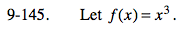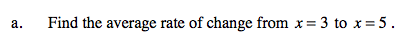$\frac{f(5)-f(3)}{5-3}$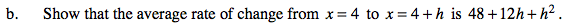$\frac{f(4+h)-f(4)}{(4+h)-4}$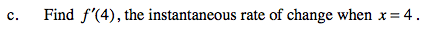$\lim_{ h \to 0} \frac{f(4+h)-f(4)}{(4+h)-4}$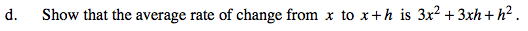$\frac{f(x+h)-f(x)}{(x+h)-x}$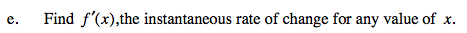$\lim_{ h \to 0}\frac{f(x+h)-f(x)}{(x+h)-x}$